•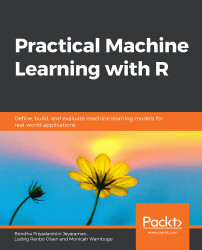#### Practical Machine Learning with R#### Overview of this book

With huge amounts of data being generated every moment, businesses need applications that apply complex mathematical calculations to data repeatedly and at speed. With machine learning techniques and R, you can easily develop these kinds of applications in an efficient way. Practical Machine Learning with R begins by helping you grasp the basics of machine learning methods, while also highlighting how and why they work. You will understand how to get these algorithms to work in practice, rather than focusing on mathematical derivations. As you progress from one chapter to another, you will gain hands-on experience of building a machine learning solution in R. Next, using R packages such as rpart, random forest, and multiple imputation by chained equations (MICE), you will learn to implement algorithms including neural net classifier, decision trees, and linear and non-linear regression. As you progress through the book, you’ll delve into various machine learning techniques for both supervised and unsupervised learning approaches. In addition to this, you’ll gain insights into partitioning the datasets and mechanisms to evaluate the results from each model and be able to compare them. By the end of this book, you will have gained expertise in solving your business problems, starting by forming a good problem statement, selecting the most appropriate model to solve your problem, and then ensuring that you do not overtrain it.Free Chapter
An Introduction to Machine LearningData Cleaning and Pre-processingFeature EngineeringIntroduction to neuralnet and Evaluation MethodsLinear and Logistic Regression ModelsUnsupervised LearningAppendix## Machine Learning Models

There are various algorithms that can be applied to numerous kinds of business problems. Broadly, the algorithms fall under supervised and unsupervised learning.

In supervised learning, the model is exposed to a set of samples with known outcomes/labels from which it learns. This process is known as training the model. After the learning/training process, the model is given a new set of data samples, based on which it performs predictions that give us the outcome. This is known as the prediction phase.

In unsupervised learning, the data samples provided to the model do not contain outcomes or labels. The model identifies patterns present in the samples and highlights the most commonly occurring patterns. Some of the approaches are clustering (hierarchical, k-means, and so on) and neural networks (self-organizing maps). This approach can also be used to detect unusual behavior in the data.

### Types of Prediction

The prediction techniques are broadly categorized into numeric prediction and categoric prediction.

Numeric prediction: When the output to be predicted is a number, it is called numeric prediction. As shown in the following example, the output is 5, 3.8, 7.6, which are numeric in nature: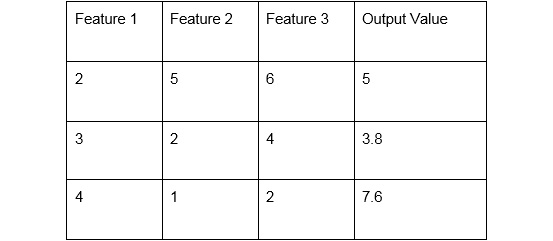###### Figure 1.30: Numeric prediction data

Categorical prediction: When the output to be predicted is a category (non-numeric), it is known as categorical prediction. It is mostly defined as a classification problem. The following data shows an example of categorical value prediction for the outputs A and G.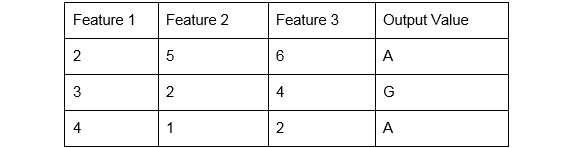###### Figure 1.31: Categorical prediction data

It is important to identify the nature of the output variable because the model should be chosen based on the type of output variable. In certain cases, the data is transformed into another type to cater to the requirements of the particular algorithm. We will now go through a list of machine learning algorithms in the following section and will discuss in detail the type of predictions they can be used for.

### Supervised Learning

Supervised learning is broadly classified as follows:

• Linear regression: This is a technique whereby the input variable and the output field are related by a linear equation, Y=aX+b. It can be implemented using the lm() function in R. This is used for predicting numerical values, for instance, predicting the revenue of a company for the next year.
• Logistic regression: This technique is used for a classification problem where the output is categorical in nature. For instance, will it rain tomorrow? The answer would be Y or N. This technique fits a function that is a closest fit of the data and is also a linear combination of the input variables. The glm() function in R is used for implementing it.
• Decision trees: A decision tree is a tree with multiple nodes and branches, where each node represents a feature and the branches from the node represent a condition for the feature value. The tree can have multiple branches, signifying multiple conditions. The leaf node of the tree is the outcome. If the outcome is continuous, it is known as a regression tree.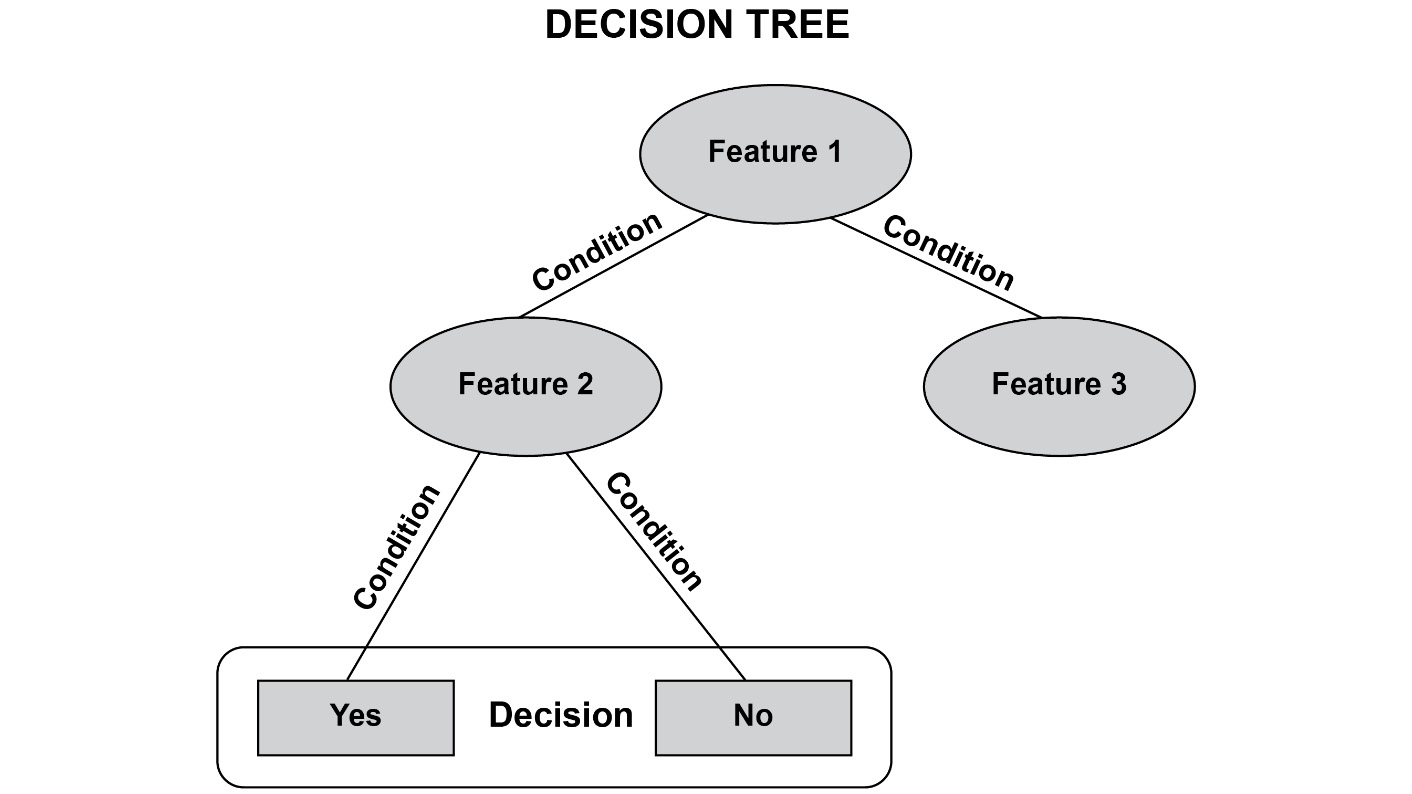###### Figure 1.32: A sample decision tree
• Support vector machines: This approach maps inputs to a higher dimensional space using a kernel function. The data is separated by hyperplanes in this higher dimensional space. The support vector machine identifies the most optimal hyperspace with a large separation. It can be used for classification problems such as categorical prediction, as well as numeric prediction. It is also known as support vector regression.
• Naïve Bayes: It is a probabilistic model that uses the Bayes' theorem to perform classification.
• Random forest: This can be used for performing classification and regression problems. It is an ensemble approach, which involves training multiple decision trees and combining the results to give the prediction.
• Neural networks: A neural network is inspired by the a human brain. It consists of interconnected neurons or nodes. It uses a backpropagation algorithm for learning. It can be used for categorical, as well as numeric, predictions.

### Unsupervised Learning

For unsupervised learning, hierarchical clustering and k-means clustering are used.

• Hierarchical clustering: The goal of a clustering process is to identify groups within the data. Each group must have data points that are very similar to each other. Also, two groups must be very different from each other in terms of their characteristics. Hierarchical clustering forms a dendrogram, a tree-like structure. There are two types of hierarchical clustering. Agglomerative clustering forms a tree-like structure in a bottom-up manner, whereas divisive hierarchical clustering forms a tree-like structure in a top-down manner.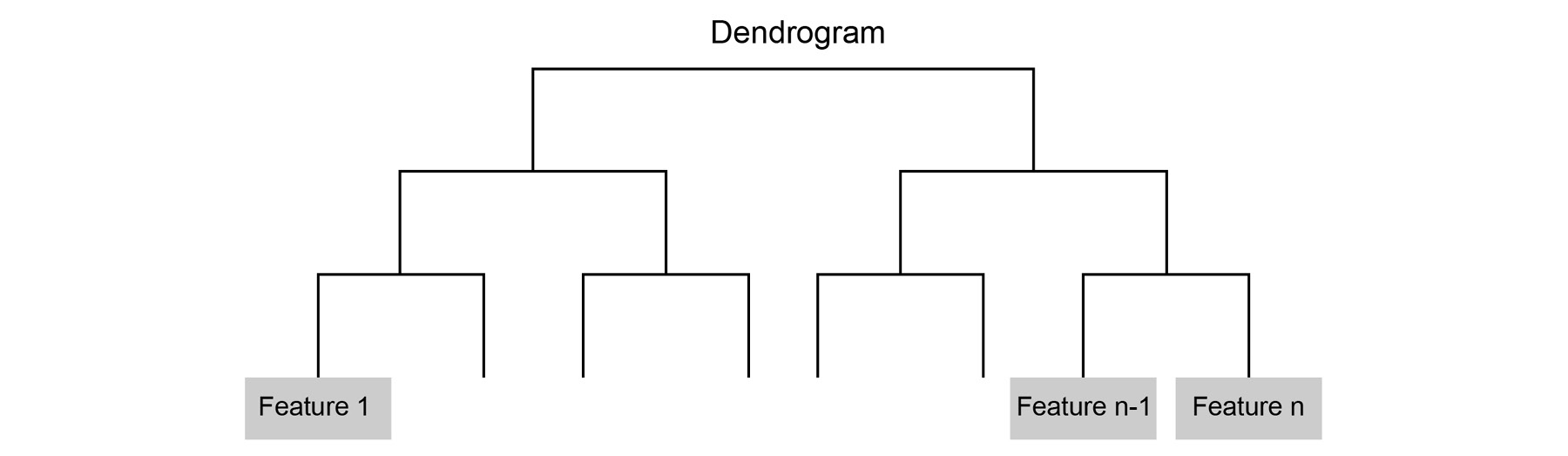###### Figure 1.33: A dendrogram
• K-means clustering: In k-means clustering, the data is grouped into k clusters. The data is grouped based on the similarity score between the data points. Here, k is a predefined value. The choice of the value determines the quality of the cluster.

### Applications of Machine Learning

The following are a few practical applications of machine learning:

• Recommendation systems: You can find a number of recommendations occurring on e-commerce websites. For instance, Amazon has several algorithms recommending products to its users. Predicting the user's next purchase or displaying products similar to the products purchased by the user are some scenarios where machine learning algorithms are used.
• Forecasting sales: In many product-based companies, the sales could be predicted for the next month/quarter/year. This can help them to better plan their resources and stocks.
• Fraud detection: Credit card fraud can be detected by using the transaction data. Classifiers can classify the fraudulent transactions, or outlier/anomaly detection can detect the anomalies in the transaction data.
• Sentiment analysis: Textual data is available in abundance. Sentiment analysis on textual data can be done using machine learning. For example, the user's sentiments can be identified as positive or negative, based on reviews of a certain product crawled from the internet. Logistic regression or Naïve Bayes can be used to identify the category using a bag of words representing the sentiments.
• Stock prediction: The stock price is predicted based on the characteristics of the stock in the past. Historical data containing the opening price, closing price, high, and low can be used.